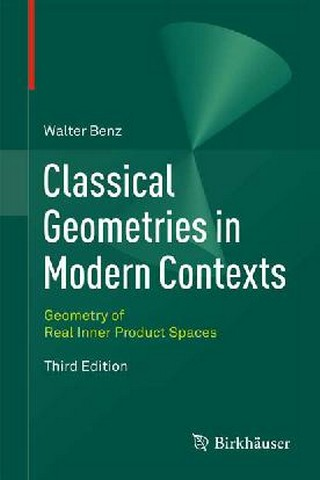### Book Toankho

Sách hay - Sách đẹp

# Classical Geometries in Modern Contexts (Geometry of Real Inner Product Spaces)The focus of this book and its geometric notions is on real vector spaces X that are finite or infinite inner product spaces of arbitrary dimension greater than or equal to 2. It characterizes both euclidean and hyperbolic geometry with respect to natural properties of (general) translations and general distances of X. Also for these spaces X, it studies the sphere geometries of Möbius and Lie as well as geometries where Lorentz transformations play the key role.
Proofs of newer theorems characterizing isometries and Lorentz transformations under mild hypotheses are included, such as for instance infinite dimensional versions of famous theorems of A.D. Alexandrov on Lorentz transformations. A real benefit is the dimension-free approach to important geometrical theories.

New to this third edition is a chapter dealing with a simple and great idea of Leibniz that allows us to characterize, for these same spaces X, hyperplanes of euclidean, hyperbolic geometry, or spherical geometry, the geometries of Lorentz-Minkowski and de Sitter, and this through finite or infinite dimensions greater than 1.

Another new and fundamental result in this edition concerns the representation of hyperbolic motions, their form and their transformations. Further we show that the geometry (P,G) of segments based on X is isomorphic to the hyperbolic geometry over X. Here P collects all x in X of norm less than one, G is defined to be the group of bijections of P transforming segments of P onto segments.

The only prerequisites for reading this book are basic linear algebra and basic 2- and 3-dimensional real geometry. This implies that mathematicians who have not so far been especially interested in geometry could study and understand some of the great ideas of classical geometries in modern and general contexts.